Bleaching of the Great Barrier Reef

22 April, 2016The chatter of gossip distracts us from the really big story, the Anthropocene: the new geological era we are bringing about. Here’s something that should be dominating the headlines: Most of the Great Barrier Reef, the world’s largest coral reef system, now looks like a ghostly graveyard.

Most corals are colonies of tiny genetically identical animals called polyps. Over centuries, their skeletons build up reefs, which are havens for many kinds of sea life. Some polyps catch their own food using stingers. But most get their food by symbiosis! They cooperate with single-celled organism called zooxanthellae. Zooxanthellae get energy from the sun’s light. They actually live inside the polyps, and provide them with food. Most of the color of a coral reef comes from these zooxanthellae.

When a polyp is stressed, the zooxanthellae living inside it may decide to leave. This can happen when the sea water gets too hot. Without its zooxanthellae, the polyp is transparent and the coral’s white skeleton is revealed—as you see here. We say the coral is bleached.

After they bleach, the polyps begin to starve. If conditions return to normal fast enough, the zooxanthellae may come back. If they don’t, the coral will die.

The Great Barrier Reef, off the northeast coast of Australia, contains over 2,900 reefs and 900 islands. It’s huge: 2,300 kilometers long, with an area of about 340,000 square kilometers. It can be seen from outer space!

With global warming, this reef has been starting to bleach. Parts of it bleached in 1998 and again in 2002. But this year, with a big El Niño pushing world temperatures to new record highs, is the worst.

Scientists have being flying over the Great Barrier Reef to study the damage, and divers have looked at some of the reefs in detail. Of the 522 reefs surveyed in the northern sector, over 80% are severely bleached and less than 1% are not bleached at all. The damage is less further south where the water is cooler—but most of the reefs are in the north: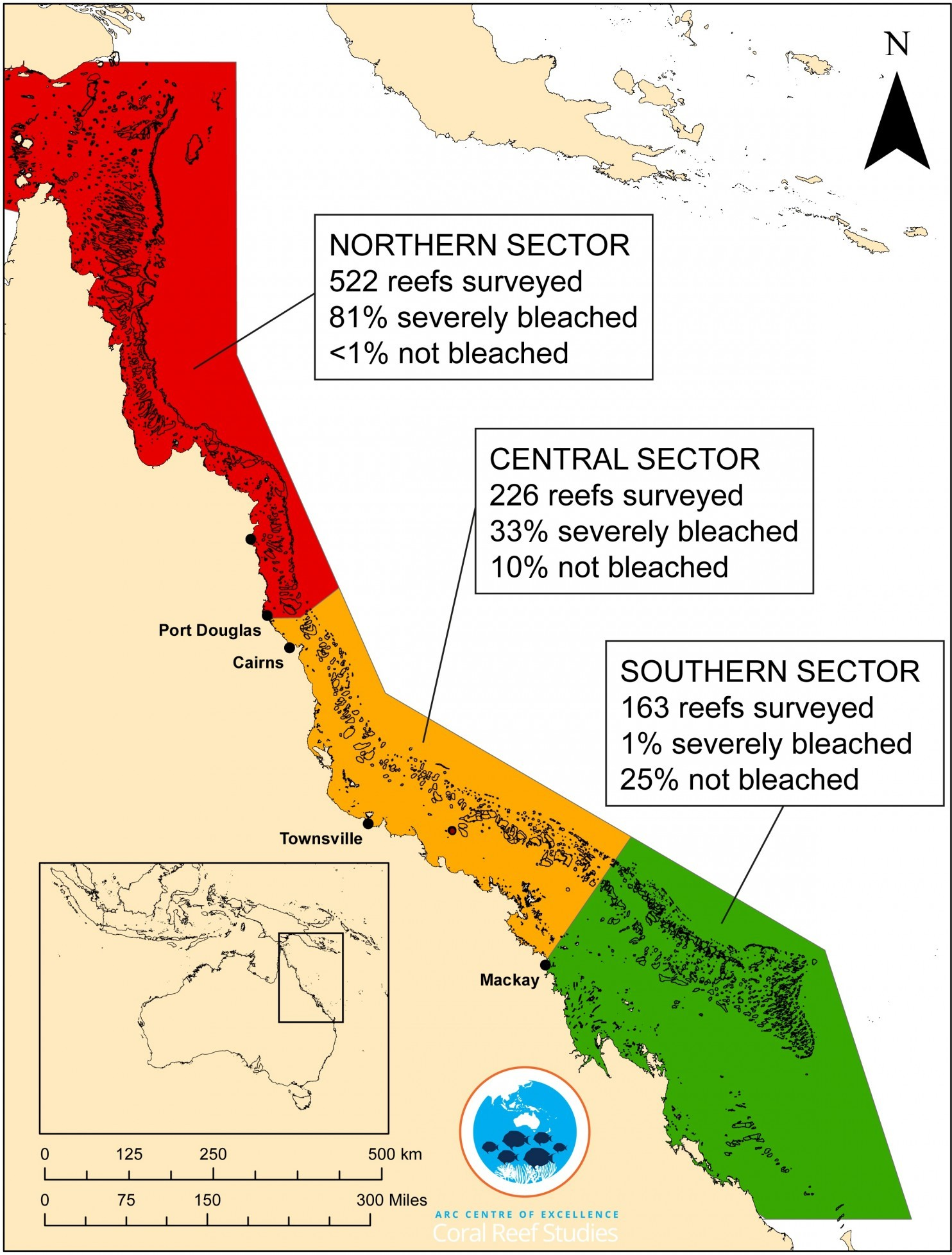The top expert on coral reefs in Australia, Terry Hughes, wrote:

I showed the results of aerial surveys of bleaching on the Great Barrier Reef to my students. And then we wept.

Imagine devoting your life to studying and trying to protect coral reefs, and then seeing this.

Some of the bleached reefs may recover. But as oceans continue to warm, the prospects look bleak. The last big El Niño was in 1998. With a lot of hard followup work, scientists showed that in the end, 16% of the world’s corals died in that event.

This year is quite a bit hotter.

So, global warming is not a problem for the future: it’s a problem now. It’s not good enough to cut carbon emissions eventually. We’ve got to get serious now.

I need to recommit myself to this. For example, I need to stop flying around to conferences. I’ve cut back, but I need to do much better. Future generations, living in the damaged world we’re creating, will not have much sympathy for our excuses.

New IPCC Report (Part 5)

14 April, 2014

guest post by Steve Easterbrook

(5) Current rates of ocean acidification are unprecedented.

The IPCC report says:

The pH of seawater has decreased by 0.1 since the beginning of the industrial era, corresponding to a 26% increase in hydrogen ion concentration. […] It is virtually certain that the increased storage of carbon by the ocean will increase acidification in the future, continuing the observed trends of the past decades. […] Estimates of future atmospheric and oceanic carbon dioxide concentrations indicate that, by the end of this century, the average surface ocean pH could be lower than it has been for more than 50 million years.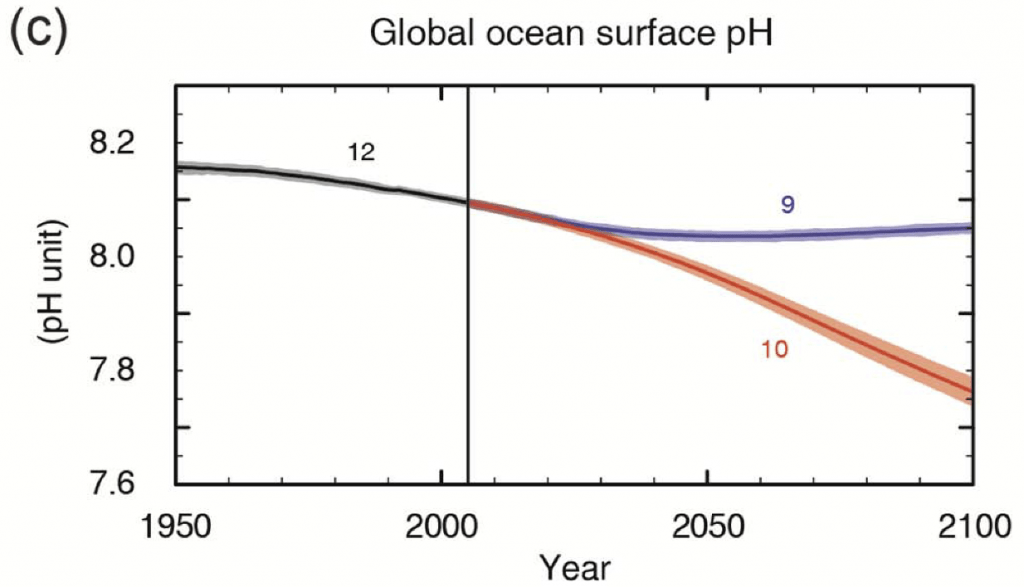(Fig SPM.7c) CMIP5 multi-model simulated time series from 1950 to 2100 for global mean ocean surface pH. Time series of projections and a measure of uncertainty (shading) are shown for scenarios RCP2.6 (blue) and RCP8.5 (red). Black (grey shading) is the modelled historical evolution using historical reconstructed forcings. [The numbers indicate the number of models used in each ensemble.]

Ocean acidification has sometimes been ignored in discussions about climate change, but it is a much simpler process, and is much easier to calculate (notice the uncertainty range on the graph above is much smaller than most of the other graphs). This graph shows the projected acidification in the best and worst case scenarios (RCP2.6 and RCP8.5). Recall that RCP8.5 is the “business as usual” future.

Note that this doesn’t mean the ocean will become acid. The ocean has always been slightly alkaline—well above the neutral value of pH 7. So “acidification” refers to a drop in pH, rather than a drop below pH 7. As this continues, the ocean becomes steadily less alkaline. Unfortunately, as the pH drops, the ocean stops being supersaturated for calcium carbonate. If it’s no longer supersaturated, anything made of calcium carbonate starts dissolving. Corals and shellfish can no longer form. If you kill these off, the entire ocean food chain is affected. Here’s what the IPCC report says:

Surface waters are projected to become seasonally corrosive to aragonite in parts of the Arctic and in some coastal upwelling systems within a decade, and in parts of the Southern Ocean within 1–3 decades in most scenarios. Aragonite, a less stable form of calcium carbonate, undersaturation becomes widespread in these regions at atmospheric CO2 levels of 500–600 ppm.

You can download all of Climate Change 2013: The Physical Science Basis here. Click below to read any part of this series:

Climate Change 2013: The Physical Science Basis is also available chapter by chapter here:

New IPCC Report (Part 4)

11 April, 2014

guest post by Steve Easterbrook

(4) Most of the heat is going into the oceans

The oceans have a huge thermal mass compared to the atmosphere and land surface. They act as the planet’s heat storage and transportation system, as the ocean currents redistribute the heat. This is important because if we look at the global surface temperature as an indication of warming, we’re only getting some of the picture. The oceans act as a huge storage heater, and will continue to warm up the lower atmosphere (no matter what changes we make to the atmosphere in the future).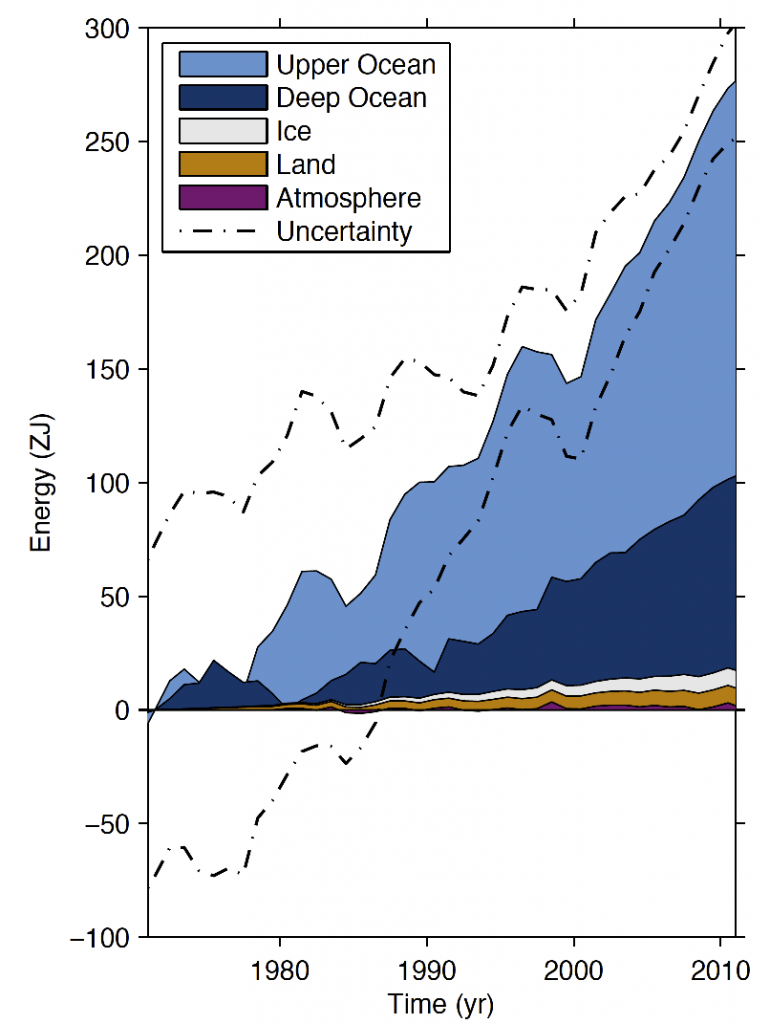(Box 3.1 Fig 1) Plot of energy accumulation in zettajoules within distinct components of Earth’s climate system relative to 1971 and from 1971–2010 unless otherwise indicated. Ocean warming (heat content change) dominates, with the upper ocean (light blue, above 700 m) contributing more than the deep ocean (dark blue, below 700 m; including below 2000 m estimates starting from 1992). Ice melt (light grey; for glaciers and ice caps, Greenland and Antarctic ice sheet estimates starting from 1992, and Arctic sea ice estimate from 1979–2008); continental (land) warming (orange); and atmospheric warming (purple; estimate starting from 1979) make smaller contributions. Uncertainty in the ocean estimate also dominates the total uncertainty (dot-dashed lines about the error from all five components at 90% confidence intervals).

Note the relationship between this figure (which shows where the heat goes) and the figure from Part 2 that showed change in cumulative energy budget from different sources: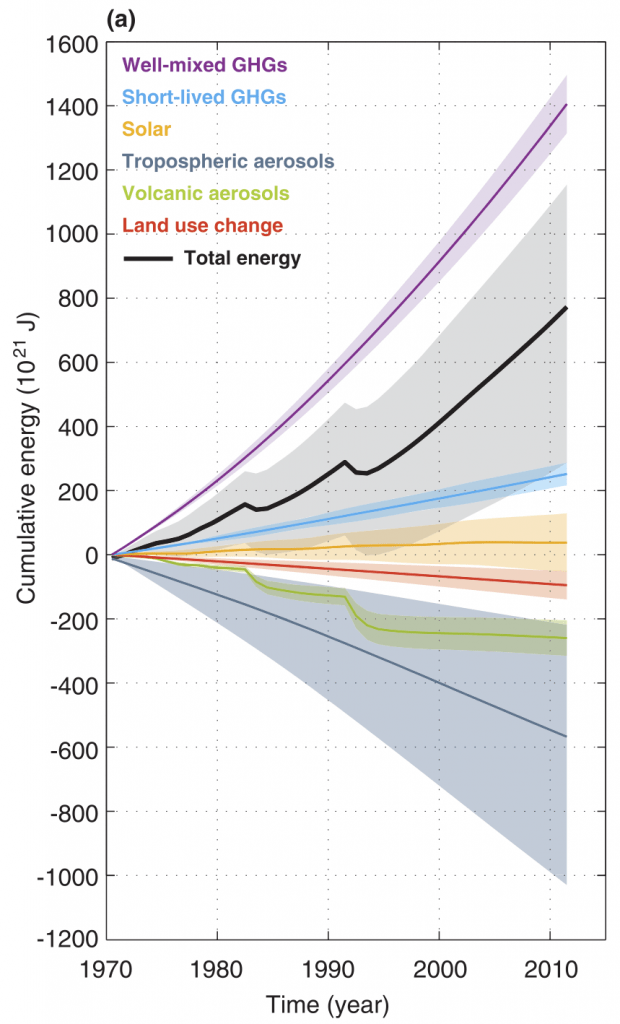(Box 13.1 fig 1) The Earth’s energy budget from 1970 to 2011. Cumulative energy flux (in zettajoules) into the Earth system from well-mixed and short-lived greenhouse gases, solar forcing, changes in tropospheric aerosol forcing, volcanic forcing and surface albedo, (relative to 1860–1879) are shown by the coloured lines and these are added to give the cumulative energy inflow (black; including black carbon on snow and combined contrails and contrail induced cirrus, not shown separately).

Both graphs show zettajoules accumulating over about the same period (1970-2011). But the graph from Part 1 has a cumulative total just short of 800 zettajoules by the end of the period, while today’s new graph shows the earth storing “only” about 300 zettajoules of this. Where did the remaining energy go? Because the earth’s temperature rose during this period, it also lost increasingly more energy back into space. When greenhouse gases trap heat, the earth’s temperature keeps rising until outgoing energy and incoming energy are in balance again.

You can download all of Climate Change 2013: The Physical Science Basis here. Click below to read any part of this series:

Climate Change 2013: The Physical Science Basis is also available chapter by chapter here:

Life’s Struggle to Survive

19 December, 2013

Here’s the talk I gave at the SETI Institute:

When pondering the number of extraterrestrial civilizations, it is worth noting that even after it got started, the success of life on Earth was not a foregone conclusion. In this talk, I recount some thrilling episodes from the history of our planet, some well-documented but others merely theorized: our collision with the planet Theia, the oxygen catastrophe, the snowball Earth events, the Permian-Triassic mass extinction event, the asteroid that hit Chicxulub, and more, including the massive environmental changes we are causing now. All of these hold lessons for what may happen on other planets!

To watch the talk, click on the video above. To see

Here’s a mistake in my talk that doesn’t appear in the slides: I suggested that Theia started at the Lagrange point in Earth’s orbit. After my talk, an expert said that at that time, the Solar System had lots of objects with orbits of high eccentricity, and Theia was probably one of these. He said the Lagrange point theory is an idiosyncratic theory, not widely accepted, that somehow found its way onto Wikipedia.

Another issue was brought up in the questions. In a paper in Science, Sherwood and Huber argued that:

Any exceedence of 35 °C for extended periods should
induce hyperthermia in humans and other mammals, as dissipation of metabolic heat becomes impossible. While this never happens now, it would begin to occur with global-mean warming of about 7 °C, calling the habitability of some regions into question. With 11-12 °C warming, such regions would spread to encompass the majority of the human population as currently distributed. Eventual warmings of 12 °C are
possible from fossil fuel burning.

However, the Paleocene-Eocene Thermal Maximum seems to have been even hotter: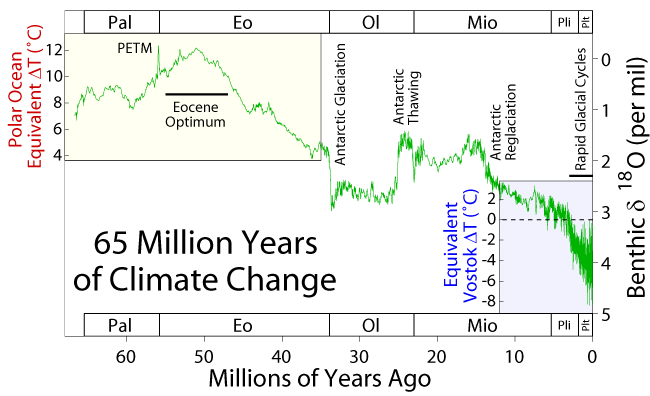So, the question is: where did mammals live during this period, which mammals went extinct, if any, and does the survival of other mammals call into question Sherwood and Huber’s conclusion?

Monte Carlo Methods in Climate Science

23 July, 2013

joint with David Tweed

One way the Azimuth Project can help save the planet is to get bright young students interested in ecology, climate science, green technology, and stuff like that. So, we are writing an article for Math Horizons, an American magazine for undergraduate math majors. This blog article is a draft of that. You can also see it in PDF form here.

We’d really like to hear your comments! There are severe limits on including more detail, since the article should be easy to read and short. So please don’t ask us to explain more stuff: we’re most interested to know if you sincerely don’t understand something, or feel that students would have trouble understanding something. For comparison, you can see sample Math Horizons articles here.

Introduction

They look placid lapping against the beach on a calm day, but the oceans are actually quite dynamic. The ocean currents act as ‘conveyor belts’, transporting heat both vertically between the water’s surface and the depths and laterally from one area of the globe to another. This effect is so significant that the temperature and precipitation patterns can change dramatically when currents do.

For example: shortly after the last ice age, northern Europe experienced a shocking change in climate from 10,800 to 9,500 BC. At the start of this period temperatures plummeted in a matter of decades. It became 7° Celsius colder, and glaciers started forming in England! The cold spell lasted for over a thousand years, but it ended as suddenly as it had begun.

Why? The most popular theory is that that a huge lake in North America formed by melting glaciers burst its bank—and in a massive torrent lasting for years, the water from this lake rushed out to the northern Atlantic ocean. By floating atop the denser salt water, this fresh water blocked a major current: the Atlantic Meridional Overturning Circulation. This current brings warm water north and helps keep northern Europe warm. So, when iit shut down, northern Europe was plunged into a deep freeze.

Right now global warming is causing ice sheets in Greenland to melt and release fresh water into the North Atlantic. Could this shut down the Atlantic Meridional Overturning Circulation and make the climate of Northern Europe much colder? In 2010, Keller and Urban [KU] tackled this question using a simple climate model, historical data, probability theory, and lots of computing power. Their goal was to understand the spectrum of possible futures compatible with what we know today.

Let us look at some of the ideas underlying their work.

Box models

The earth’s physical behaviour, including the climate is far too complex to simulate from the bottom up using basic physical principles, at least for now. The most detailed models today can take days to run on very powerful computers. So to make reasonable predictions on a laptop in a tractable time-frame, geophysical modellers use some tricks.

First, it is possible to split geophysical phenomena into ‘boxes’ containing strongly related things. For example: atmospheric gases, particulate levels and clouds all affect each other strongly; likewise the heat content, currents and salinity of the oceans all interact strongly. However, the interactions between the atmosphere and the oceans are weaker, and we can approximately describe them using just a few settings, such as the amount of atmospheric CO2 entering or leaving the oceans. Clearly these interactions must be consistent—for example, the amount of CO2 leaving the atmosphere box must equal the amount entering the ocean box—but breaking a complicated system into parts lets different specialists focus on different aspects; then we can combine these parts and get an approximate model of entire planet. The box model used by Keller and Urban is shown in Figure 1.1. The box model used by Keller and Urban.

Second, it turn out that simple but effective box models can be distilled from the complicated physics in terms of forcings and feedbacks. Essentially a forcing is a measured input to the system, such as solar radiation or CO2 released by burning fossil fuels. As an analogy, consider a child on a swing: the adult’s push every so often is a forcing. Similarly a feedback describes how the current ‘box variables’ influence future ones. In the swing analogy, one feedback is how the velocity will influence the future height. Specifying feedbacks typically uses knowledge of the detailed low-level physics to derive simple, tractable functional relationships between groups of large-scale observables, a bit like how we derive the physics of a gas by thinking about collisions of lots of particles.

However, it is often not feasible to get actual settings for the parameters in our model starting from first principles. In other words, often we can get the general form of the equations in our model, but they contain a lot of constants that we can estimate only by looking at historical data.

Probability modeling

Suppose we have a box model that depends on some settings$S.$ For example, in Keller and Urban’s model,$S$ is a list of 18 numbers. To keep things simple, suppose the settings are element of some finite set. Suppose we also have huge hard disc full of historical measurements, and we want to use this to find the best estimate of$S.$ Because our data is full of ‘noise’ from other, unmodeled phenomena we generally cannot unambiguously deduce a single set of settings. Instead we have to look at things in terms of probabilities. More precisely, we need to study the probability that$S$ take some value$s$ given that the measurements take some value. Let’s call the measurements$M$, and again let’s keep things simple by saying$M$ takes values in some finite set of possible measurements.

The probability that$S = s$ given that$M$ takes some value$m$ is called the conditional probability$P(S=s | M=m).$ How can we compute this conditional probability? This is a somewhat tricky problem.

One thing we can more easily do is repeatedly run our model with randomly chosen settings and see what measurements it predicts. By doing this, we can compute the probability that given setting values$S = s,$ the model predicts measurements$M=m.$ This again is a conditional probability, but now it is called$P(M=m|S=s).$

This is not what we want: it’s backwards! But here Bayes’ rule comes to the rescue, relating what we want to what we can more easily compute:$\displaystyle{ P(S = s | M = m) = P(M = m| S = s) \frac{P(S = s)}{P(M = m)} }$

Here$P(S = s)$ is the probability that the settings take a specific value$s,$ and similarly for$P(M = m).$ Bayes’ rule is quite easy to prove, and it is actually a general rule that applies to any random variables, not just the settings and the measurements in our problem [Y]. It underpins most methods of figuring out hidden quantities from observed ones. For this reason, it is widely used in modern statistics and data analysis [K].

How does Bayes’ rule help us here? When we repeatedly run our model with randomly chosen settings, we have control over$P(S = s).$ As mentioned, we can compute$P(M=m| S=s).$ Finally,$P(M = m)$ is independent of our choice of settings. So, we can use Bayes’ rule to compute$P(S = s | M = m)$ up to a constant factor. And since probabilities must sum to 1, we can figure out this constant.

This lets us do many things. It lets us find the most likely values of the settings for our model, given our hard disc full of observed data. It also lets us find the probability that the settings lie within some set. This is important: if we’re facing the possibility of a climate disaster, we don’t just want to know the most likely outcome. We would like to know to know that with 95% probability, the outcome will lie in some range.

An example

Let us look at an example much simpler than that considered by Keller and Urban. Suppose our measurements are real numbers$m_0,\dots, m_T$ related by$m_{t+1} = s m_t - m_{t-1} + N_t$

Here$s,$ a real constant, is our ‘setting’, while$N_t$ is some ‘noise’: an independent Gaussian random variable for each time$t,$ each with mean zero and some fixed standard deviation. Then the measurements$m_t$ will have roughly sinusoidal behavior but with irregularity added by the noise at each time step, as illustrated in Figure 2.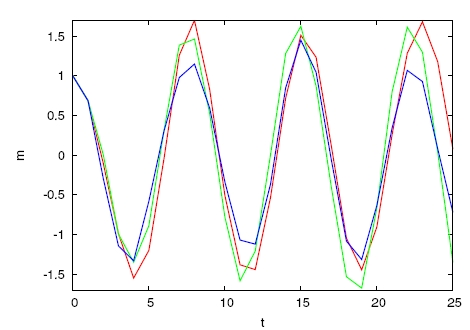2. The example system: red are predicted measurements for a given value of the settings, green is another simulation for the same$s$ value and blue is a simulation for a slightly different$s.$

Note how there is no clear signal from either the curves or the differences that the green curve is at the correct setting value while the blue one has the wrong one: the noise makes it nontrivial to estimate$s.$ This is a baby version of the problem faced by Keller and Urban.

Markov Chain Monte Carlo

Having glibly said that we can compute the conditional probability$P(M=m | S=s),$ how do we actually do this? The simplest way would be to run our model many, many times with the settings set at$S=s$ and determine the fraction of times it predicts measurements equal to$m.$ This gives us an estimate of$P(M=m | S=s).$ Then we can use Bayes’ rule to work out$P(M=m|S=s),$ at least up to a constant factor.

Doing all this by hand would be incredibly time consuming and error prone, so computers are used for this task. In our example, we do this in Figure 3. As we keep running our model over and over, the curve showing$P(M=m |S=s)$ as a function of$s$ settles down to the right answer.3. The estimates of$P(M=m | S=s)$ as a function of$s$ using uniform sampling, ending up with 480 samples at each point.

However, this is computationally inefficient, as shown in the probability distribution for small numbers of samples. This has quite a few ‘kinks’, which only disappear later. The problem is that there are lots of possible choices of$s$ to try. And this is for a very simple model!

When dealing with the 18 settings involved in the model of Keller and Urban, trying every combination would take far too long. A way to avoid this is Markov Chain Monte Carlo sampling. Monte Carlo is famous for its casinos, so a ‘Monte Carlo’ algorithm is one that uses randomness. A ‘Markov chain’ is a random walk: for example, where you repeatedly flip a coin and take one step right when you get heads, and one step right when you get tails. So, in Markov Chain Monte Carlo, we perform a random walk through the collection of all possible settings, collecting samples.

The key to making this work is that at each step on the walk a proposed modification$s'$ to the current settings$s$ is generated randomly—but it may be rejected if it does not seem to improve the estimates. The essence of the rule is:

The modification$s \mapsto s'$ is randomly accepted with a probability equal to the ratio$\displaystyle{ \frac{P(M=m | S=s')}{ P(M=m | S=s)} }$

Otherwise the walk stays at the current position.

If the modification is better, so that the ratio is greater than 1, the new state is always accepted. With some additional tricks—such as discarding the very beginning of the walk—this gives a set of samples from which can be used to compute$P(M=m | S=s).$ Then we can compute$P(S = s | M = m)$ using Bayes’ rule.

Figure 4 shows the results of using the Markov Chain Monte Carlo procedure to figure out$P(S= s| M= m)$ in our example.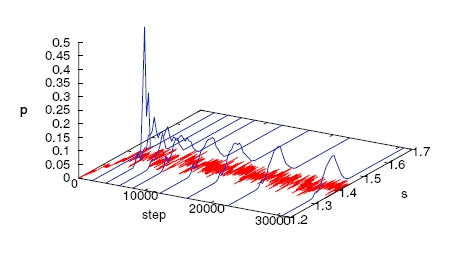4. The estimates of$P(S = s|M = m)$ curves using Markov Chain Monte Carlo, showing the current distribution estimate at increasing intervals. The red line shows the current position of the random walk. Again the kinks are almost gone in the final distribution.

Note that the final distribution has only peformed about 66 thousand simulations in total, while the full sampling peformed over 1.5 million. The key advantage of Markov Chain Monte Carlo is that it avoids performing many simulations in areas where the probability is low, as we can see from the way the walk path remains under the big peak in the probability density almost all the time. What is more impressive is that it achieves this without any global view of the probability density, just by looking at how$P(M=m | S=s)$ changes when we make small changes in the settings. This becomes even more important as we move to dealing with systems with many more dimensions and settings, where it proves very effective at finding regions of high probability density whatever their shape.

Why is it worth doing so much work to estimate the probability distribution for settings for a climate model? One reason is that we can then estimate probabilities of future events, such as the collapse of the Atlantic Meridional Ocean Current. And what’s the answer? According to Keller and Urban’s calculation, this current will likely weaken by about a fifth in the 21st century, but a complete collapse is unlikely before 2300. This claim needs to be checked in many ways—for example, using more detailed models. But the importance of the issue is clear, and we hope we have made the importance of good mathematical ideas for climate science clear as well.

Exploring the topic

The Azimuth Project is a group of scientists, engineers and computer programmers interested in questions like this [A]. If you have questions, or want to help out, just email us. Versions of the computer programs we used in this paper will be made available here in a while.

Here are some projects you can try, perhaps with the help of Kruschke’s textbook [K]:

• There are other ways to do setting estimation using time series: compare some to MCMC in terms of accuracy and robustness.

• We’ve seen a 1-dimensional system with one setting. Simulate some multi-dimensional and multi-setting systems. What new issues arise?

Acknowledgements. We thank Nathan Urban and other
members of the Azimuth Project for many helpful discussions.

References

[A] Azimuth Project, http://www.azimuthproject.org.

[KU] Klaus Keller and Nathan Urban, Probabilistic hindcasts and projections of the coupled climate, carbon cycle and Atlantic meridional overturning circulation system: a Bayesian fusion of century-scale measurements with a simple model, Tellus A 62 (2010), 737–750. Also available free online.

[K] John K. Kruschke, Doing Bayesian Data Analysis: A Tutorial with R and BUGS, Academic Press, New York, 2010.

[Y] Eliezer S. Yudkowsky, An intuitive explanation of Bayes’ theorem.

Petri Net Programming (Part 2)

20 December, 2012

guest post by David A. Tanzer

An introduction to stochastic Petri nets

In the previous article, I explored a simple computational model called Petri nets. They are used to model reaction networks, and have applications in a wide variety of fields, including population ecology, gene regulatory networks, and chemical reaction networks. I presented a simulator program for Petri nets, but it had an important limitation: the model and the simulator contain no notion of the rates of the reactions. But these rates critically determine the character of the dynamics of network.

Here I will introduce the topic of ‘stochastic Petri nets,’ which extends the basic model to include reaction dynamics. Stochastic means random, and it is presumed that there is an underlying random process that drives the reaction events. This topic is rich in both its mathematical foundations and its practical applications. A direct application of the theory yields the rate equation for chemical reactions, which is a cornerstone of chemical reaction theory. The theory also gives algorithms for analyzing and simulating Petri nets.

We are now entering the ‘business’ of software development for applications to science. The business logic here is nothing but math and science itself. Our study of this logic is not an academic exercise that is tangential to the implementation effort. Rather, it is the first phase of a complete software development process for scientific programming applications.

The end goals of this series are to develop working code to analyze and simulate Petri nets, and to apply these tools to informative case studies. But we have some work to do en route, because we need to truly understand the models in order to properly interpret the algorithms. The key questions here are when, why, and to what extent the algorithms give results that are empirically predictive. We will therefore be embarking on some exploratory adventures into the relevant theoretical foundations.

The overarching subject area to which stochastic Petri nets belong has been described as stochastic mechanics in the network theory series here on Azimuth. The theme development here will partly parallel that of the network theory series, but with a different focus, since I am addressing a computationally oriented reader. For an excellent text on the foundations and applications of stochastic mechanics, see:

• Darren Wilkinson, Stochastic Modelling for Systems Biology, Chapman and Hall/CRC Press, Boca Raton, Florida, 2011.

Review of basic Petri nets

A Petri net is a graph with two kinds of nodes: species and transitions. The net is populated with a collection of ‘tokens’ that represent individual entities. Each token is attached to one of the species nodes, and this attachment indicates the type of the token. We may therefore view a species node as a container that holds all of the tokens of a given type.

The transitions represent conversion reactions between the tokens. Each transition is ‘wired’ to a collection of input species-containers, and to a collection of output containers. When it ‘fires’, it removes one token from each input container, and deposits one token to each output container.

Here is the example we gave, for a simplistic model of the formation and dissociation of H2O molecules: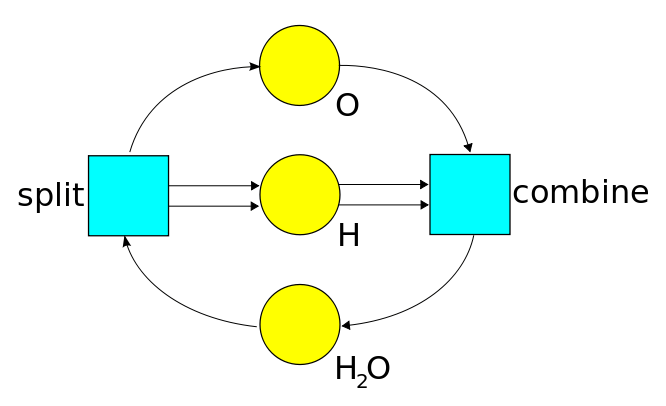The circles are for species, and the boxes are for transitions.

The transition combine takes in two H tokens and one O token, and outputs one H2O token. The reverse transition is split, which takes in one H2O, and outputs two H’s and one O.

An important application of Petri nets is to the modeling of biochemical reaction networks, which include the gene regulatory networks. Since genes and enzymes are molecules, and their binding interactions are chemical reactions, the Petri net model is directly applicable. For example, consider a transition that inputs one gene G, one enzyme E, and outputs the molecular form G • E in which E is bound to a particular site on G.

Applications of Petri nets may differ widely in terms of the population sizes involved in the model. In general chemistry reactions, the populations are measured in units of moles (where a mole is ‘Avogadro’s number’ 6.022 · 1023 entities). In gene regulatory networks, on the other hand, there may only be a handful of genes and enzymes involved in a reaction.

This difference in scale leads to a qualitative difference in the modelling. With small population sizes, the stochastic effects will predominate, but with large populations, a continuous, deterministic, average-based approximation can be used.

Representing Petri nets by reaction formulas

Petri nets can also be represented by formulas used for chemical reaction networks. Here is the formula for the Petri net shown above:

H2O ↔ H + H + O

or the more compact:

H2O ↔ 2 H + O

The double arrow is a compact designation for two separate reactions, which happen to be opposites of each other.

By the way, this reaction is not physically realistic, because one doesn’t find isolated H and O atoms traveling around and meeting up to form water molecules. This is the actual reaction pair that predominates in water:

2 H2O ↔ OH + H3O+

Here, a hydrogen nucleus H+, with one unit of positive charge, gets removed from one of the H2O molecules, leaving behind the hydroxide ion OH. In the same stroke, this H+ gets re-attached to the other H2O molecule, which thereby becomes a hydronium ion, H3O+.

For a more detailed example, consider this reaction chain, which is of concern to the ocean environment:

CO2 + H2O ↔ H2CO3 ↔ H+ + HCO3

This shows the formation of carbonic acid, namely H2CO3, from water and carbon dioxide. The next reaction represents the splitting of carbonic acid into a hydrogen ion and a negatively charged bicarbonate ion, HCO3. There is a further reaction, in which a bicarbonate ion further ionizes into an H+ and a doubly negative carbonate ion CO32-. As the diagram indicates, for each of these reactions, a reverse reaction is also present. For a more detailed description of this reaction network, see:

• Stephen E. Bialkowski, Carbon dioxide and carbonic acid.

Increased levels of CO2 in the atmosphere will change the balance of these reactions, leading to a higher concentration of hydrogen ions in the water, i.e., a more acidic ocean. This is of concern because the metabolic processes of aquatic organisms is sensitive to the pH level of the water. The ultimate concern is that entire food chains could be disrupted, if some of the organisms cannot survive in a higher pH environment. See the Wikipedia page on ocean acidification for more information.

Exercise. Draw Petri net diagrams for these reaction networks.

Motivation for the study of Petri net dynamics

The relative rates of the various reactions in a network critically determine the qualitative dynamics of the network as a whole. This is because the reactions are ‘competing’ with each other, and so their relative rates determine the direction in which the state of the system is changing. For instance, if molecules are breaking down faster then they are being formed, then the system is moving towards full dissociation. When the rates are equal, the processes balance out, and the system is in an equilibrium state. Then, there are only temporary fluctuations around the equilibrium conditions.

The rate of the reactions will depend on the number of tokens present in the system. For example, if any of the input tokens are zero, then the transition can’t fire, and so its rate must be zero. More generally, when there are few input tokens available, there will be fewer reaction events, and so the firing rates will be lower.

Given a specification for the rates in a reaction network, we can then pose the following kinds of questions about its dynamics:

• Does the network have an equilibrium state?

• If so, what are the concentrations of the species at equilibrium?

• How quickly does it approach the equilibrium?

• At the equilibrium state, there will still be temporary fluctuations around the equilibrium concentrations. What are the variances of these fluctuations?

• Are there modes in which the network will oscillate between states?

This is the grail we seek.

Aside from actually performing empirical experiments, such questions can be addressed either analytically or through simulation methods. In either case, our first step is to define a theoretical model for the dynamics of a Petri net.

Stochastic Petri nets

A stochastic Petri net (with kinetics) is a Petri net that is augmented with a specification for the reaction dynamics. It is defined by the following:

• An underlying Petri net, which consists of species, transitions, an input map, and an output map. These maps assign to each transition a multiset of species. (Multiset means that duplicates are allowed.) Recall that the state of the net is defined by a marking function, that maps each species to its population count.

• A rate constant that is associated with each transition.

• A kinetic model, that gives the expected firing rate for each transition as a function of the current marking. Normally, this kinetic function will include the rate constant as a multiplicative factor.

A further ‘sanity constraint’ can be put on the kinetic function for a transition: it should give a positive value if and only if all of its inputs are positive.

• A stochastic model, which defines the probability distribution of the time intervals between firing events. This specific distribution of the firing intervals for a transition will be a function of the expected firing rate in the current marking.

This definition is based on the standard treatments found, for example in:

• M. Ajmone Marsan, Stochastic Petri nets: an elementary introduction, in Advances in Petri Nets, Springer, Berlin, 1989, 1–23.

or Wilkinson’s book mentioned above. I have also added an explicit mention of the kinetic model, based on the ‘kinetics’ described in here:

• Martin Feinberg, Lectures on chemical reaction networks.

There is an implied random process that drives the reaction events. A classical random process is given by a container with ‘particles’ that are randomly traveling around, bouncing off the walls, and colliding with each other. This is the general idea behind Brownian motion. It is called a random process because the outcome results from an ‘experiment’ that is not fully determined by the input specification. In this experiment, you pour in the ingredients (particles of different types), set the temperature (the distributions of the velocities), give it a stir, and then see what happens. The outcome consists of the paths taken by each of the particles.

In an important limiting case, the stochastic behavior becomes deterministic, and the population sizes become continuous. To see this, consider a graph of population sizes over time. With larger population sizes, the relative jumps caused by the firing of individual transitions become smaller, and graphs look more like continuous curves. In the limit, we obtain an approximation for high population counts, in which the graphs are continuous curves, and the concentrations are treated as continuous magnitudes. In a similar way, a pitcher of sugar can be approximately viewed as a continuous fluid.

This simplification permits the application of continuous mathematics to study of reaction network processes. It leads to the basic rate equation for reaction networks, which specifies the direction of change of the system as a function of the current state of the system.

In this article we will be exploring this continuous deterministic formulation of Petri nets, under what is known as the mass action kinetics. This kinetics is one implementation of the general specification of a kinetic model, as defined above. This means that it will define the expected firing rate of each transition, in a given marking of the net. The probabilistic variations in the spacing of the reactions—around the mean given by the expected firing rate—is part of the stochastic dynamics, and will be addressed in a subsequent article.

The mass-action kinetics

Under the mass action kinetics, the expected firing rate of a transition is proportional to the product of the concentrations of its input species. For instance, if the reaction were A + C → D, then the firing rate would be proportional to the concentration of A times the concentration of C, and if the reaction were A + A → D, it would be proportional to the square of the concentration of A.

This principle is explained by Feinberg as follows:

For the reaction A+C → D, an occurrence requires that a molecule of A meet a molecule of C in the reaction, and we take the probability of such an encounter to be proportional to the product [of the concentrations of A and C]. Although we do not presume that every such encounter yields a molecule of D, we nevertheless take the occurrence rate of A+C → D to be governed by [the product of the concentrations].

For an in-depth proof of the mass action law, see this article:

• Daniel Gillespie, A rigorous definition of the chemical master equation, 1992.

Note that we can easily pass back and forth between speaking of the population counts for the species, and the concentrations of the species, which is just the population count divided by the total volume V of the system. The mass action law applies to both cases, the only difference being that the constant factors of (1/V) used for concentrations will get absorbed into the rate constants.

The mass action kinetics is a basic law of empirical chemistry. But there are limits to its validity. First, as indicated in the proof in the Gillespie, the mass action law rests on the assumptions that the system is well-stirred and in thermal equilibrium. Further limits are discussed here:

• Georg Job and Regina Ruffler, Physical Chemistry (first five chapters), Section 5.2, 2010.

They write:

…precise measurements show that the relation above is not strictly adhered to. At higher concentrations, values depart quite noticeably from this relation. If we gradually move to lower concentrations, the differences become smaller. The equation here expresses a so-called “limiting law“ which strictly applies only when c → 0.

In practice, this relation serves as a useful approximation up to rather high concentrations. In the case of electrically neutral substances, deviations are only noticeable above 100 mol m−3. For ions, deviations become observable above 1 mol m−3, but they are so small that they are easily neglected if accuracy is not of prime concern.

Why would the mass action kinetics break down at high concentrations? According to the book quoted, it is due to “molecular and ionic interactions.” I haven’t yet found a more detailed explanation, but here is my supposition about what is meant by molecular interactions in this context. Doubling the number of A molecules doubles the number of expected collisions between A and C molecules, but it also reduces the probability that any given A and C molecules that are within reacting distance will actually react. The reaction probability is reduced because the A molecules are ‘competing’ for reactions with the C molecules. With more A molecules, it becomes more likely that a C molecule will simultaneously be within reacting distance of several A molecules; each of these A molecules reduces the probability that the other A molecules will react with the C molecule. This is most pronounced when the concentrations in a gas get high enough that the molecules start to pack together to form a liquid.

The equilibrium relation for a pair of opposite reactions

Suppose we have two opposite reactions:$T: A + B \stackrel{u}{\longrightarrow} C + D$$T': C + D \stackrel{v}{\longrightarrow} A + B$

Since the reactions have exactly opposite effects on the population sizes, in order for the population sizes to be in a stable equilibrium, the expected firing rates of$T$ and$T'$ must be equal:$\mathrm{rate}(T') = \mathrm{rate}(T)$

By mass action kinetics:$\mathrm{rate}(T) = u [A] [B]$$\mathrm{rate}(T') = v [C] [D]$

where$[X]$ means the concentration of$X.$

Hence at equilibrium:$u [A] [B] = v [C] [D]$

So:$\displaystyle{ \frac{[A][B]}{[C][D]} = \frac{v}{u} = K }$

where$K$ is the equilibrium constant for the reaction pair.

Equilibrium solution for the formation and dissociation of a diatomic molecule

Let A be some type of atom, and let D = A2 be the diatomic form of A. Then consider the opposite reactions:$A + A \stackrel{u}{\longrightarrow} D$$D \stackrel{v}{\longrightarrow} A + A$

From the preceding analysis, at equilibrium the following relation holds:$u [A]^2 = v [D]$

Let$N(A)$ and$N(B)$ be the population counts for A and B, and let$N = N(A) + 2 N(D)$

be the total number of units of A in the system, whether they be in the form of atoms or diatoms.

The value of$N$ is an invariant property of the system. The reactions cannot change it, because they are just shuffling the units of A from one arrangement to the other. By way of contrast,$N(A)$ is not an invariant quantity.

Dividing this equation by the total volume$V$, we get:$[N] = [A] + 2 [D]$

where$[N]$ is the concentration of the units of A.

Given a fixed value for$[N]$ and the rate constants$u$ and$v$, we can then solve for the concentrations at equilibrium:$\displaystyle{u [A]^2 = v [D] = v ([N] - [A]) / 2 }$$\displaystyle{2 u [A]^2 + v [A] - v [N] = 0 }$$\displaystyle{[A] = (-v \pm \sqrt{v^2 + 8 u v [N]}) / 4 u }$

Since$[A]$ can’t be negative, only the positive square root is valid.

Here is the solution for the case where$u = v = 1$:$\displaystyle{[A] = (\sqrt{8 [N] + 1} - 1) / 4 }$$\displaystyle{[D] = ([N] - [A]) / 2 }$

Conclusion

We’ve covered a lot of ground, starting with the introduction of the stochastic Petri net model, followed by a general discussion of reaction network dynamics, the mass action laws, and calculating equilibrium solutions for simple reaction networks.

We still have a number of topics to cover on our journey into the foundations, before being able to write informed programs to solve problems with stochastic Petri nets. Upcoming topics are (1) the deterministic rate equation for general reaction networks and its application to finding equilibrium solutions, and (2) an exploration of the stochastic dynamics of a Petri net. These are the themes that will support our upcoming software development.

Tsunami

12 March, 2011

I hope everyone reading this, and everyone they know, is okay…

Stories, anyone?

Check out this animation from NOAA, the National Oceanic and Atmospheric Administration:

The tsunami was unnoticeable here in Singapore. It was just 10 centimeters tall when it hit the North Maluku islands in Indonesia, and we’re protected from the open Pacific by lots of Indonesian islands.

Of course, this “protection” has its own dangers, since Indonesia is geologically active: since I’ve lived here there have been two volcanic eruptions in Java, and an earthquake in western Sumatra created a tsunami that killed over 282 people in the Mentawai islands. An earthquake in eastern Sumatra could cause a tsunami here, perhaps—Sumatra is visible from tall buildings downtown. But today things are fine, here.

They’re worse in California!—though as you might expect, some there took advantage of the tsunami for surfing.